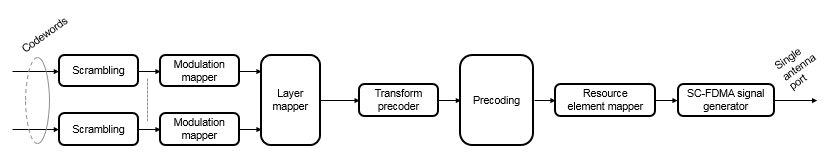# ltePSSCHPRBS

PSSCH pseudorandom binary scrambling sequence

## Syntax

``[seq,cinit] = ltePSSCHPRBS(ue,n)``
``[seq,cinit] = ltePSSCHPRBS(ue,n,mapping)``
``[subseq,cinit] = ltePSSCHPRBS(ue,pn)``
``[subseq,cinit] = ltePSSCHPRBS(ue,pn,mapping)``

## Description

example

````[seq,cinit] = ltePSSCHPRBS(ue,n)` returns the first `n` outputs of the PSSCH pseudorandom binary scrambling sequence (PRBS) for the specified UE settings structure. It also returns an initialization value `cinit` for the pseudorandom binary sequence (PRBS) generator.The scrambling sequence generated should be applied to the coded PSSCH data carried by the associated subframe. The PRBS sequence generator used is initialized with ${c}_{\text{init}}={n}_{\text{ID}}^{\text{X}}×{2}^{14}+{n}_{\text{ssf}}^{\text{PSSCH}}×{2}^{9}+510$. For more information, see Physical Sidelink Shared Channel Processing, `ue`.`NSAID` and `ue`.`NXID````

example

````[seq,cinit] = ltePSSCHPRBS(ue,n,mapping)` specifies the format of the returned sequence, `seq`, through the `mapping` input.```
````[subseq,cinit] = ltePSSCHPRBS(ue,pn)` returns a subsequence of a full PRBS sequence, specified by `pn`.```
````[subseq,cinit] = ltePSSCHPRBS(ue,pn,mapping)` specifies the format of the returned subsequence, `subseq`, through the `mapping` input.```

## Examples

collapse all

Scramble a PSSCH codeword by generating the PSSCH pseudorandom binary sequence (PRBS) and applying an exclusive OR operation on the two sequences.

Create a UE settings structure with required fields. Generate the required length of the PRBS. Scramble the PSSCH codeword with the PRBS sequence using `xor`.

```ue = struct('NSAID',255,'NSubframePSSCH',0); codeword = ones(1152,1); psschPrbs = ltePSSCHPRBS(ue,length(codeword)); scrambled = xor(psschPrbs,codeword);```

Scramble PSSCH Codeword

• Generate the required length of the PRBS.

• Scramble the PSSCH codeword with the PRBS sequence using `xor`.

• Modulate the logical scrambled data.

```ue = struct('NSAID',255,'NSubframePSSCH',0); codeword = ones(1152,1); psschPrbs = ltePSSCHPRBS(ue,length(codeword)); scrambled = xor(psschPrbs,codeword); txsym = lteSymbolModulate(scrambled,'16QAM');```

Descramble Recovered Codeword

• Generate the PSSCH PRBS in signed form.

• Descramble the vector representing a sequence of soft bits by generating the PSSCH PRBS in signed form and performing a pointwise multiplication between the PRBS sequence and the recovered soft data.

• Compare the transmitted codeword to the recovered codeword.

```sym = awgn(txsym,30,'measured'); softdata = lteSymbolDemodulate(sym,'16QAM'); scramblingSeq = ltePSSCHPRBS(ue,length(softdata),'signed'); descrambled = softdata.*scramblingSeq; isequal(codeword,descrambled > 0)```
```ans = logical 1 ```

The transmitted codeword matches the hard decision on the descrambled data.

## Input Arguments

collapse all

User equipment settings, specified as a parameter structure containing these fields:

Sidelink mode, specified as `'D2D'` or `'V2X'`.

Data Types: `char` | `string`

Sidelink group destination identity, specified as an integer in the interval [0, 255].

This field is the lower eight bits of the full 24-bit ProSe Layer-2 group destination ID. This field and the `NSubframePSSCH` field control the value of the scrambling sequence at the start of each subframe. This field is required only for D2D sidelink.

Data Types: `double`

V2X scrambling identity, specified as an integer scalar. `NXID` is the 16 bit CRC associated with the PSCCH SCI grant. It is only required for V2X sidelink.

Data Types: `double`

PSSCH subframe number in the PSSCH subframe pool, specified as an integer scalar. (${n}_{\text{ssf}}^{\text{PSSCH}}$)

`NSubframePSSCH` and `NSAID` control the values of the scrambling sequence. It is only required for D2D sidelink.

Data Types: `double`

Data Types: `struct`

Number of elements in returned sequence, `seq`, specified as a numeric scalar.

Data Types: `double`

Range of elements in returned subsequence, `subseq`, specified as a row vector of `[p n]`. The subsequence returns `n` values of the PRBS generator, starting at position `p` (0-based).

Data Types: `double`

Output sequence formatting, specified as `'binary'` or `'signed'`.

• `'binary'` maps `true` to 1 and `false` to 0.

• `'signed'` maps `true` to –1 and `false` to 1.

Data Types: `char` | `string`

## Output Arguments

collapse all

PSSCH pseudorandom scrambling sequence, returned as a logical column vector or a numeric column vector. `seq` contains the first `n` outputs of the physical sidelink shared channel (PSSCH) scrambling sequence. If you set `mapping` to `'signed'`, the output data type is `double`. Otherwise, the output data type is `logical`.

Data Types: `logical` | `double`

PSSCH pseudorandom scrambling subsequence, returned as a logical column vector or a numeric column vector. `subseq` contains the values of the PRBS generator specified by `pn`. If you set `mapping` to `'signed'`, the output data type is `double`. Otherwise, the output data type is `logical`.

Data Types: `logical` | `double`

Initialization value for PRBS generator, returned as a numeric scalar.

Data Types: `uint32`

collapse all

### Physical Sidelink Shared Channel Processing

Physical sidelink shared channel (PSSCH) processing includes PSSCH-specific scrambling, QPSK or 16-QAM modulation, and SC-FDMA transform precoding. PSSCH processing follows the processing steps used for PUSCH, with variations defined in TS 36.211, Section 9.3.For PSSCH, the input codeword length is Mbits = NRE × Nbps, where Nbps is the number of bits per symbol. PSSCH modulation is either QPSK (2 bits per symbol) or 16 QAM (4 bits per symbol).

The number of PSSCH resource elements (NRE) in a subframe is NRE = NPRB × NREperPRB × NSYM and includes symbols associated with the sidelink SC-FDMA guard symbol.

• NPRB is the number of physical resource blocks (PRB) used for transmission.

• NREperPRB is the number of resource elements in a PRB. Each PRB has 12 resource elements.

• NSYM is the number of SC-FDMA symbols in a PSSCH subframe, including symbols associated with the sidelink SC-FDMA guard symbol. The number of SC-FDMA symbols in a PSSCH subframe is 12 for D2D normal cyclic prefix or 10 for D2D extended cyclic prefix and V2X.

The `info` structure output by `ltePSSCHIndices` provides Mbits and NRE as `info.G` and `info.Gd` respectively.

The scrambling sequence generator is initialized with ${c}_{\text{init}}={n}_{\text{ID}}^{\text{X}}×{2}^{14}+{n}_{\text{ssf}}^{\text{PSSCH}}×{2}^{9}+510$ at the start of every PSSCH subframe. For D2D sidelink, ${n}_{\text{ID}}^{\text{SA}}$ is the destination identity (`NSAID`) obtained from the sidelink shared channel. For V2X, ${n}_{\text{ID}}^{\text{SA}}$ is the V2X scrambling identity (`NXID`). ${n}_{\text{ssf}}^{\text{PSSCH}}$ is the subframe number in the PSSCH subframe pool (`NSubframePSSCH`).

`ltePSSCH` requires `CyclicPrefixSL` to deduce the number of resource blocks allocated for SC-FDMA precoding symbols.

 3GPP TS 36.211. “Evolved Universal Terrestrial Radio Access (E-UTRA); Physical Channels and Modulation.” 3rd Generation Partnership Project; Technical Specification Group Radio Access Network. URL: https://www.3gpp.org.